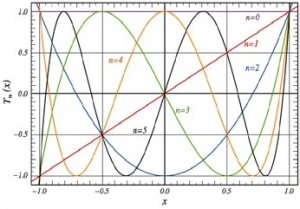## Research faculty members in this area

### Approximation theory

Approximation theory studies how one can approximate general, possibly complicated, functions/curves/surfaces, etc. by simpler and more easily calculated objects. For instance, the Weierstrass approximation theorem shows that any continuous function can be uniformly approximated by polynomials (which are infinitely smooth), while Weierstrass himself constructed an example of a nowhere differentiable continuous function. In modern approximation theory, a variety of tools, algorithms and methods are available, which are used in different areas of analysis (e.g., in harmonic analysis and Fourier analysis) and mathematics (e.g., foundations for numerical methods), and also have very practical applications such as image compression, signal processing, curve and surface fitting.

Shape-preserving approximation is a type of constrained approximation: we demand that the approximating tool preserves certain geometric properties of the function, such as a sign, monotonicity, convexity, etc. Normally the element of best-unconstrained approximation is oscillating around the target function, but such oscillation may be undesired in applications, so specialized methods need to be developed. Shape-preserving requirements appear naturally in some problems of computer-aided geometric design. Research focuses on how much of the accuracy of approximation we must sacrifice in order to fulfill shape constraints.

One of the main tasks of approximation theory is to investigate relations between the smoothness of the approximated function and the error of approximation. If a function is “smoother” (e.g. has more derivatives), one can usually achieve a better approximation rate. Conversely, the magnitude of the approximation error carries information about the smoothness of the function. To achieve such direct and converse results, one needs to properly measure the smoothness of the function, and derivatives are usually not sensitive enough for the task. One of the applications of measures of smoothness is the analysis of the speed of numerical solutions of integral and partial differential equations.### Your future in approximation theory

Students in approximation theory can find jobs in academia and industry. Many of our MSc graduates continue their studies at the PhD level in various prestigious universities both in Canada and abroad (the University of Alberta, University of British Columbia, University of Montreal, Max Planck Institute for Mathematics in Bonn), and our PhD graduates have been successful in finding jobs in academia or industry.